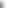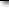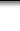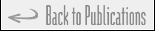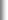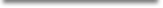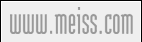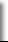# Progressive Interval Heuristics for Multi-Item Capacitated Lot Sizing Problems## Awi Federgruen, Joern Meissner, Michal TzurAbstractWe consider a family of N items which are produced in or obtained from the same production facility. Demands are deterministic for each item and each period within a given horizon of T periods. If in a given period an order is placed, setup costs are incurred. The aggregate order size is constrained by a capacity limit. The objective is to find a lot-sizing strategy that satisfies the demands for all items over the entire horizon without backlogging, and which minimizes the sum of inventory carrying, fixed and variable order costs. All demands, cost parameters and capacity limits may be time-dependent. In the basic (JS)-model, the setup cost of an order does not depend on the composition of the order. The (JIS)-model allows for item-dependent setup costs in addition to the joint setup costs.

We develop and analyze a class of so-called progressive interval heuristics. A progessive interval heuristic solves a (JS) or (JIS) problem over a progressively larger time-interval, always starting with period 1, but fixing the setup variables of a progressively larger number of periods at their optimal values in earlier iterations. Different variants in this class of heuristics allow for different degrees of flexibility in adjusting continuous variables determined in earlier iterations of the algorithm.

For the (JS)-model and the two basic implementations of the progressive interval heuristics, we show under some mild parameter conditions, that the heuristics can be designed to be epsilon-optimal for any desired value of epsilon > 0 with a running time that is polynomially bounded in the size of the problem. They can also be designed to be simultaneously asymptotically optimal and polynomially bounded.

A numerical study covering both the (JS) and the (JIS) model, shows that a progressive interval heuristic generates close-to-optimal solutions with modest computational effort and that it can be effectively used to solve large-scale problems.Keywords### supply chain management, inventory models, lot sizing, time partitioningJournalOperations Research Vol 55, No 3 (May–June 2007), pp 490–502.Downloadwww.meiss.com/download/SC-Federgruen-Meissner-Tzur.pdf
(186 kb)ReferenceBibTeX, Plain Text